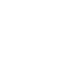# SAT Question of the Day (ACT too!): Feb. 19, 2014

If you are reading this in an email you received from me, do not click the link to sat.collegeboard.org below. Use the link to my website that is farther down on the email.

http://sat.collegeboard.org/practice/sat-question-of-the-day?questionId=20140219&oq=1 (This link takes you to today’s question. If you use my archive, you will see the question related to my SAT explanation for that date.)

The answer is C.  Start with the sentence’s topic.  What does it tell us about the subject of the sentence, the “actor’s performance?”  It was very moving and moved people to tears.  So that we don’t get attracted by the distractors (wrong answers), let’s make a prediction for the blank.  I chose “deeply affecting” because I like to use words from the sentence because they must be consistent with the topic of the sentence.  I also could have predicted “emotional.”

Only about half of the students are getting this question correct; so let’s pretend I don’t know the vocabulary and I don’t recognize the right answer.  Let’s see how many words I recognize well enough to know they do not mean “emotional” or  “deeply affecting.”  I bet most students know Answers A, D, and E: negligible, amiable, and receptive.  Let’s throw them out.  Now the big question.  How do we choose between B and C, insipid and poignant?  Don’t randomly guess!  Read the main part of the sentence and plug in the two remaining answers.  One will sound better than the other and you probably don’t even know why.  Pick the word that seems better.  This strategy will not give you a 100% correct response but it will improve your guessing above 50%.  That will raise your score.

I wonder what the ACT folks have up their sleeves this morning!

The answer is C.  Recognizing the good, old slope-intercept equation answers this question.  The coefficient of x is 3 and the constant is 1.  That means the slope is 3 and the y-intercept is 1 which gives us Answer C.  But read on because it is not as important for you to get this question correct as it is to see what you can learn about the ACT and SAT tests by doing it.

This is not a typical way the slope-intercept equation is tested.  This question is too straight forward.  More often than not, you will see a slope-intercept equation in a non-standard form such as x = (y – 1)/3 which is the same equation that is in this morning’s question.  Then they may ask, “Which of the following equations is parallel to this equation on the coordinate grid?”  You need to restate (Pillar II) the question as, “Which equation has the same slope?”  That is the signal that this equation is a slope-intercept and you need to solve for y so that you have a coefficient for x.  Then find the answer that has a slope of positive 3 and you are all done.

Have a great day.  It is “hump day;” so it is all downhill from here.

The SAT & ACT Wizard